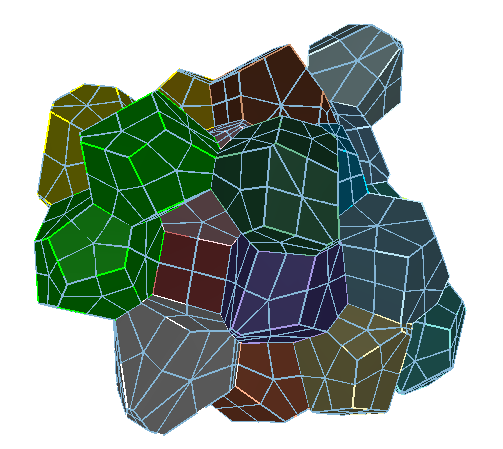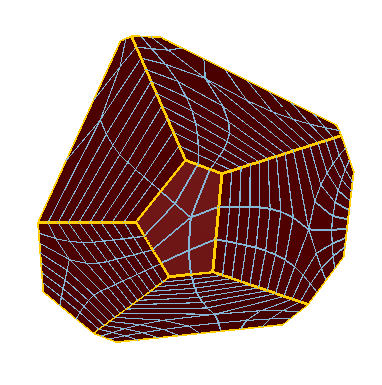# Polyhedron

Applies to: Surfaces and Volumes.

Summary: Produces an arbitrary-sided block primitive mesh for a surface or volume.

Syntax:

Volume <range> Scheme Polyhedron

Surface <range> Scheme Polyhedron

Discussion:

The polyhedron scheme is a meshing primitive for 2d and 3d n-sided regions. This is similar to the triprimitive , tetprimitive, and pentagon schemes, except rather than 3, 4, or 5 sides, it allows an arbitrary number of sides. The scheme works best on convex regions. Surfaces must have only one loop, and each vertex must be connected to exactly two curves on the surface (e.g., no hardlines). Volumes must have only one shell, each vertex must be connected to exactly three surfaces on the volume, and each surface should be meshed with scheme polyhedron. There are some interval assignment requirements as well, which should be automatically handled by CUBIT.

If the polyhedron scheme is specified for the volume, then the surfaces of the volume are automatically assigned scheme polyhedron as well, unless they were hard-set by the user. Schemes should be specified on all volumes of an assembly prior to meshing any of them. Scheme polyhedron attaches extra data to volumes; if Cubit is behaving strangely, the user may need to explicitly remove that data with a reset volume all, or similar command.

Scheme polyhedron was designed for assemblies of material grains, where each volume is roughly a Voronoi region, and the assembly is a periodic space-filling model (tile). Figure 1 shows two examples of polyhedron meshes.Figure 1. Examples of Polyhedron Scheme Meshes